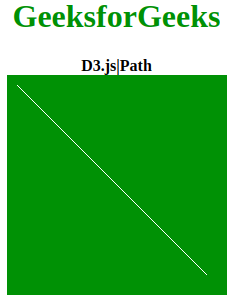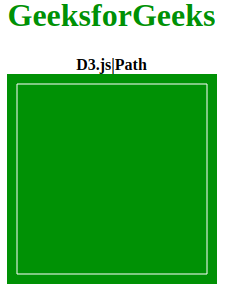Open in App
Not now

# D3.js | Path

• Last Updated : 22 Jun, 2020

D3.js is mostly used for making of graph and visualizing data on the HTML SVG elements. D3 somehow is related to Data-Driven Documents. The Path  is used to make the SVG , Path create a object that has all properties of canvas PATH. This library is also capable of drawing simulations, 2D graphs, and 3D graphs. Projections are also an inbuilt feature of this library.

Syntax:

`path.`

Parameters: There are no parameters or arguments are required.

Return: This Path returns an object that has the same method of path as provided by HTML canvas.

Example 1:

## HTML

 ```<``html` `lang``=``"en"``>``   ``<``head``>``      ``<``meta` `charset``=``"UTF-8"``>``      ``<``meta` `name``=``"viewport"` `            ``path1tent="``width``=``device``-width, ``                       ``initial-scale``=``1``.0">``      ``<``title``>D3.js| Path``   ````   ``<``style``>``      ``body {``          ``text-align: center;``      ``}``      ``h1 {``          ``color: green;``      ``}``      ``svg{``      ``background-color: green;``      ``}``      ``.path1{``      ``fill: aliceblue;``      ``}``   ````   ``<``body``>``      ``<``h1``>GeeksforGeeks``      ``<``b``>D3.js|Path``      ``<``div``>``         ``<``svg` `width``=``"220"` `height``=``"220"``>``            ``<``path` `class``=``"path1"` `stroke``=``"white"``>``         ````      ````      ``<``script` `src` `= ``"https://d3js.org/d3.v4.min.js"``>``      ``<``script``>``         ``var path = d3.path();``          ` `         ``// Starting points are x:10 and y:10``         ``path.moveTo(10, 10); ``          ` `         ``// Making line to points x:200 and y:200``         ``path.lineTo(200, 200); ``         ``d3.select(".path1").attr("d",path)``      ````   `````

Output:Example 2:

## HTML

 ```<``html` `lang``=``"en"``>``   ``<``head``>``      ``<``meta` `charset``=``"UTF-8"``>``      ``<``meta` `name``=``"viewport"` `            ``path1tent="``width``=``device``-width, ``                       ``initial-scale``=``1``.0">``      ``<``title``>D3.js| Path``   ````   ``<``style``>``      ``body {``          ``text-align: center;``      ``}``      ``h1 {``          ``color: green;``      ``}``      ``svg{``      ``background-color: green;``      ``}``      ``.path1{``      ``fill: aliceblue;``      ``}``   ````   ``<``body``>``      ``<``h1``>GeeksforGeeks``      ``<``b``>D3.js|Path``      ``<``div``>``         ``<``svg` `width``=``"210"` `height``=``"210"``>``            ``<``path` `class``=``"path1"` `stroke``=``"white"``>``         ````      ````      ``<``script` `src` `= ``"https://d3js.org/d3.v4.min.js"``>``      ``<``script``>``         ``var path = d3.path();``         ``// Point start at x:10 y:10``         ``path.moveTo(10, 10);  ``          ` `         ``// Making line to x:10 y:200``         ``path.lineTo(10, 200); ``          ` `         ``// Point start at x:10 y:10``         ``path.moveTo(10, 200); ``          ` `         ``// Making line to x:200 y:200``         ``path.lineTo(200, 200); ``          ` `         ``// Point start at x:200 y:200``         ``path.moveTo(200, 200); ``          ` `         ``// Making line to x:200 y:10``         ``path.lineTo(200, 10);``          ` `         ``// Point start at x:200 y:10``         ``path.moveTo(200, 10); ``          ` `         ``// Making line to x:10 y:10``         ``path.lineTo(10, 10);  ``         ``d3.select(".path1").attr("d",path)``      ````   `````

Output:My Personal Notes arrow_drop_up